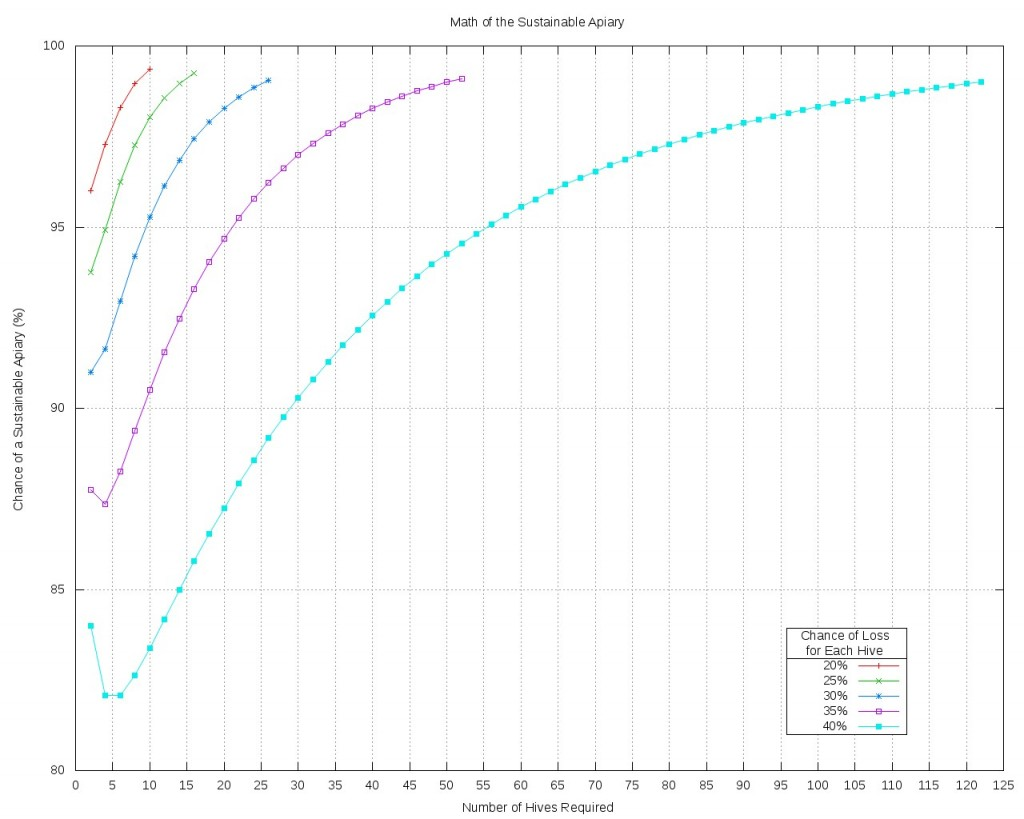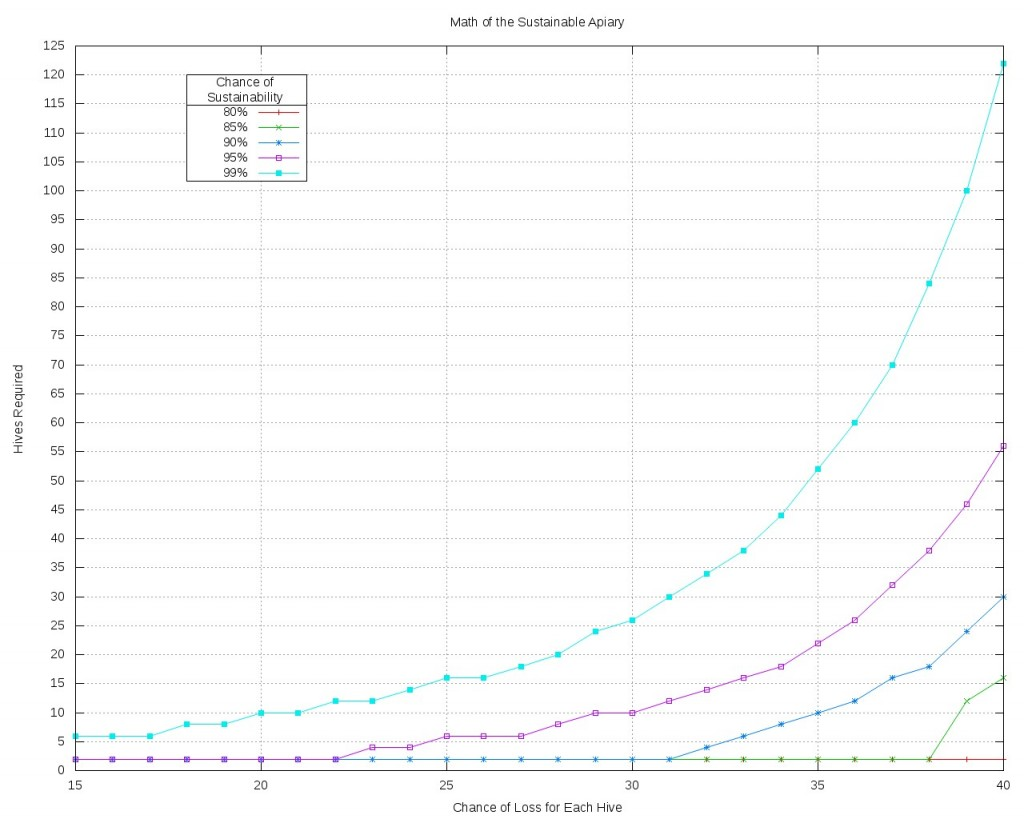As I wrote in an earlier post, the idea of a sustainable apiary has been making the rounds as a new sort of goal for which to shoot.  In that earlier post I wondered how possible this was, and how many hives I’d need to keep to achieve it.  This felt like a math problem.  The requirement for probability, statistics, and a lot of graphing visuals were all there.  Here are the results of all of those grey matter firings.

First let me state that I wanted this work to give me a direction and to give me usable information.  But I did not need it to unify gravity with quantum mechanics.  Long story short…keep it simple, and don’t go nuts with the math.  Correctness counts, complexity not so much.

If I was to apply math to this issue, I first had to define the issue mathematically.  What is the end result of a sustainable apiary in mathetmatical terms.  Because I’m in the southern end of the northern winters, and that is when we lose our bees, I defined a sustainable apiary as one where over time, one would lose no more than half his colonies each winter.  This would allow the beekeeper, in the worst of years, to split every remaining hive, and return to his original number.

Of course with any chance of loss at all, there’s always a chance of losing more than half, just as there’s always a chance of losing all hives.  If we treat this problem as a binomial distribution though, where each hive is an independent trial with a certain probability that it will die or succeed, we can then also assign a probability to at least half of the hives surviving.  This idea, using a binomial distribution, is how I tackled the problem.

In our problem we have three variables.

1. Number of Hives
2. Probability of Loss for Each Hive
3. Chance of Success for a Sustainable Apiary

Success here is defined as I wrote above.  This is the probabilty that no more than half the hives will die.  We can tweak any two of these variables to find the third.  Here are some examples:

• In order to have a 95% chance of success, meaning a 95% chance of no more than half the hives dying, and the probabilty of each hive dying is 30%, how many hives does one need?
• Having 10 hives and a 35% chance of loss, what will be the chance of success?
• With 15 hives and desiring a 98% chance of success, what loss rate is acceptable in order to achieve that success rate?

Because our question was phrased as “no more than” 1/2 the hives dying, the problem was a sum of probabilities, or in other words, a sum of a many separate calculations.  This is long and drawn out work, so statistics books normally have a table to look up these answers.  In my case I had a Perl script that I wrote.

The other key regarding this problem is how to visualize this data.  A table would work, but I felt this just wouldn’t get the point across.  A graph would do much more and allow me to see the trend vs just reading the numbers.

So without furthur ado, here are the graphs that I generated with a few comments below each.What this graph shows is that for each loss rate, we need an increasingly large number of hives in order to have what I consider a decent shot at reaching a sustainable apiary.  If I’m going to have this goal, I don’t want an 80% chance of reaching it.  That would mean I’m operating with a 20% chance of failure in reaching my goal.  I want at least a 95% chance of success.  Any less than that would just not be worth my effort.

So let’s focus on the area above the top horizontal grid line which corresponds to a 95% chance of success.  As the loss rate increases, so does the number of hives required in order to achieve that success.  But what’s astounding is the rate of the increase.  If my loss rate is 40%, I need 56 hives in order to get a 95% chance of sucess.  56!  If I can lower that loss rate by a mere 5%, down to 35%, I only need about 22 hives to achieve the same result.  Take the losses down another 5% and I only need 10 hives.  So what this graph tells me is that every single percentage point I can knock off the chance of loss is important, every single percent is worthwhile.

Let’s look at the data in a slightly different way.This graph shows us how many hives we need but allows us to pick both our chance of success and the loss rate first and just follow the curve.  The points are just slightly different than the first graph in that each point here is done in whole numbers because our y variable is discrete, we cannot have less than a whole of a hive.

I said that my own minimum chance of success that I would accept is 95%.  That’s the purple curve.  According to this data, I can achieve that with only 2 hives up until my loss percentage goes above 22%.  Once I hit 30% loss, I now need 10 hives to achieve that.  But let’s assume I have loftier goals.  What if I want to have a 99% chance of success.  I know that right now, 10 hives would be difficult to manage, that would be my upper limit for now.  That means I need to get my loss percentage down to 20% if I want to achieve that 99% chance of success number…a lofty goal indeed.

The other fact I glean from these graphs is not necessarily specific to precise numbers.  It’s an overarching theme that goes back to that earlier post.  Can a hobbyist beekeeper achieve a sustainable apiary, as I have defined it, with 2 hives, with 3, with 4?  The numbers bear out that the only way for a beekeeper to achieve this with what I consider a reasonable degree of success is for him to reduce the loss percentage as much as possible, and every point counts.  The other method of course, is to have a lot of hives.  If the losses are up in the mid 30% range, then no, a sustainable apiary is not achievable, for all intents and purposes, with any less than about 25 hives.  If this count is too high, as it is for me, then the choice is clear.  Get those loss percentages down.  Do everything to lower it even one or two points, and this could make all the difference.

You’ll notice I bolded the “as I have defined it” above.  I did that because the numbers and graphs only apply to my definition.  If you define a sustainable apiary differently, then you’ll have to do your own math, but for me right now, this definiton is good, and the data, while being a little hard to swallow, is easy to understand.